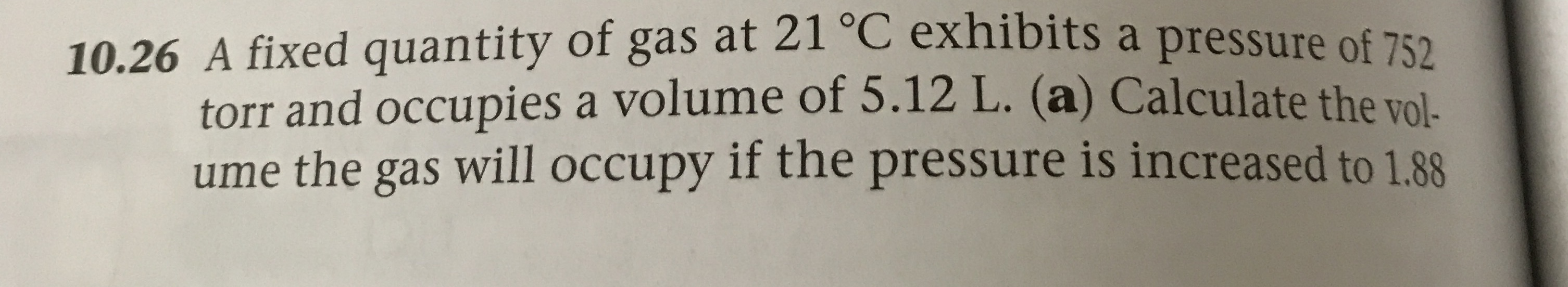# 10.26 A fixed quantity of gas at 21 C exhibits a pressure of 752torr and occupies a volume of 5.12 L. (a) Calculate the vol-ume the gas will occupy if the pressure is increased to 1.88

Question
5 viewshelp_outlineImage Transcriptionclose10.26 A fixed quantity of gas at 21 C exhibits a pressure of 752 torr and occupies a volume of 5.12 L. (a) Calculate the vol- ume the gas will occupy if the pressure is increased to 1.88 fullscreen
check_circle

Step 1

Given:

Initial Volume V1 = 5.12 L.

Initial Pressure P1 = 752 torr = 0.9895 atm

Temperature does not change.

Step 2

Ideal gas equation is given by:

Step 3

Calculation for Volume V2:

Pressure increased to 1.88 times of initial pressure

Final...

### Want to see the full answer?

See Solution

#### Want to see this answer and more?

Solutions are written by subject experts who are available 24/7. Questions are typically answered within 1 hour.*

See Solution
*Response times may vary by subject and question.
Tagged in

### States of matter